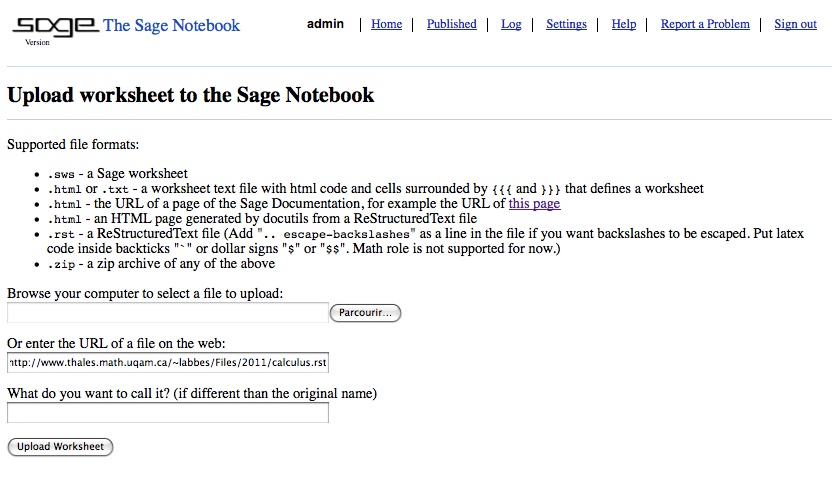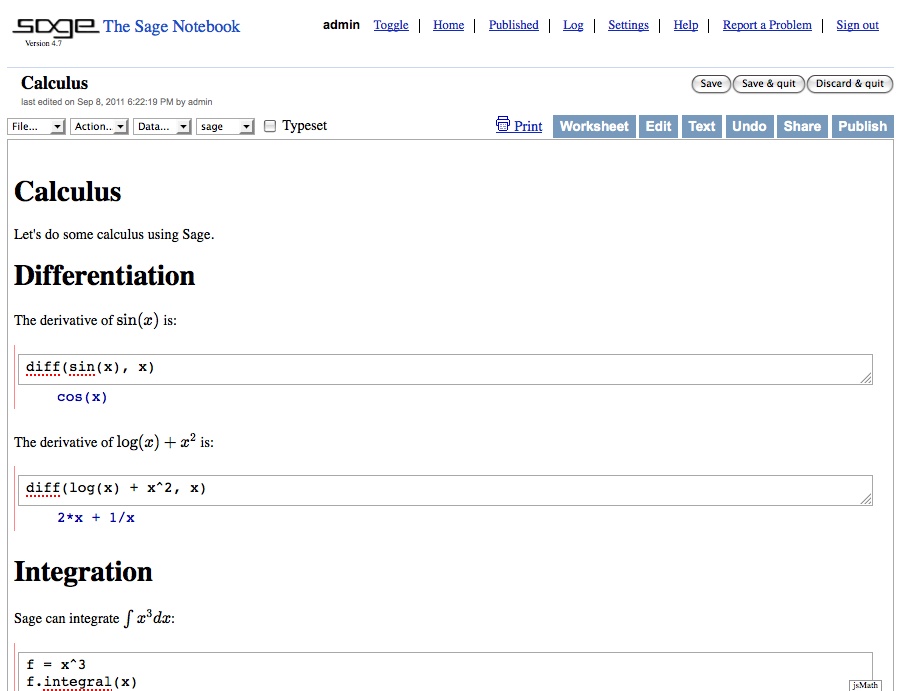ReST to Sage Worksheet

## ReST to Sage Worksheet

17 juin 2011 | Mise à jour: 08 septembre 2011 | Catégories: rst, sage | View Comments

During Sage Days 31, I worked on the ticket #11459 to implement rst to sws conversion. This post intends to give some documentation about this new feature. Here is an example of a ReStructuredText file calculus.rst:

********
Calculus
********

Let's do some calculus using Sage.

Differentiation
===============

The derivative of $\\sin(x)$ is::

sage: diff(sin(x), x)
cos(x)

The derivative of $\\log(x) + x^2$ is::

sage: diff(log(x) + x^2, x)
2*x + 1/x

Integration
===========

Sage can integrate $\\int x^3 dx$::

sage: f = x^3
sage: f.integral(x)
1/4*x^4

Let's compute $\\int x \\sin(x^2) dx$::

sage: f = x*sin(x^2)
sage: integral(f,x)
-1/2*cos(x^2)


Both the files calculus.rst and calculus.html, which was generated from the file calculus.rst using Docutils and the command rst2html.py calculus.rst calculus.html, can be uploaded into the Sage Notebook (copy their URL):This will create a new worksheet:I also implemented two command line scripts to generate the worksheet text file:

sage -rst2txt file.rst file.txt


and to generate the Sage worksheet (.sws) directly:

sage -rst2sws file.rst file.sws


I also added the possibility to automatically escape every backslashes if they are not already so that they don't get lost in the translation process. For more info, consult the documentation:

sage -rst2txt -h
sage -rst2sws -h